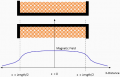# Solenoid coil ampere-turns

#### Momentory

Joined Sep 4, 2022
67
Since more turns = increase in wire lenght = increase in resistance = decrease in current

So how come more coil turns will increase the strength of electromagnetic field?!

Let Say i have a coil wire gauge = 18 Awg of lenght 100m

And an other coil wire = 16 Awg of lenght 70m

Which will produce more force?

#### Momentory

Joined Sep 4, 2022
67
Up to the point where the magnetic components saturate you can assume the force is proportional to the product of amps and turns. When you use thinner wire and more turns the coil resistance will increase so for a given voltage the current will decrease. Hence wire diameter and number of turns are optimised for a specified voltage. The force is theoretically limited by the point where the magnetic components saturate and in practice when the coil gets too hot!

Is there is any refence for wire diameter and number of turns specs for specific voltage?

#### DickCappels

Joined Aug 21, 2008
9,505
For a given fixed current force is proportional to Turns X Ampers.

#### MisterBill2

Joined Jan 23, 2018
13,689
Since more turns = increase in wire lenght = increase in resistance = decrease in current

So how come more coil turns will increase the strength of electromagnetic field?!

Let Say i have a coil wire gauge = 18 Awg of lenght 100m

And an other coil wire = 16 Awg of lenght 70m

Which will produce more force?
The magnetic force is proportional to the product of current and turns. In ythisexample the increase in resistance is quite small, so the decrease in current will be quite small. But the increase in turns is quite large, and so while the current will be a bit less the turns are a lot more, and so the magnetic field will be greater. This is assuming that the applied voltage is the same. So it is ALWAYS IMPORTANT to consider the effect of all of the variables when evaluating changes. Consider also that the smaller wire will be more compact and thus alow a few more turns than just the increase in length will produce.

#### panic mode

Joined Oct 10, 2011
2,360
Let Say i have a coil wire gauge = 18 Awg of lenght 100m

And an other coil wire = 16 Awg of lenght 70m

Which will produce more force?
mixing wire gage with metric unit of length? tsk tsk...
the first one will have resistance of about 1.9 Ohm
the second one will be about 0.84 Ohm

so if they are both powered from same source (same voltage) current in thicker and shorter wire will be larger (about 2x).
but the thinner wire is not twice as long. you do the math.

on the other hand, thinner wire may be used to make coil with smaller diameter (and more turns) and guess what happens there...

#### DickCappels

Joined Aug 21, 2008
9,505
From memory, in a perfectly wound layered coil using circular wire 37% of the winding area is empty (not copper). Then along came the people who make wire with a rectangular or square cross section or that is a long enamel insulated spiral strip.

#### DickCappels

Joined Aug 21, 2008
9,505
As I said, I that was from memory. I worked out a long time ago.

During the 1980's there was a lot of creativity poured into making switching power supply transformers more efficient. No,I was not testing you.

•Jerry-Hat-Trick

#### Danko

Joined Nov 22, 2017
1,487
So how come more coil turns will increase the strength of electromagnetic field?!
Your coil, when accurately wound by #18 wire, has these parameters.
When coil is used in such circuit, then amplitude of magnetic field is 1.288 tesla.

For #16 wire you easily can:
recalculate coil parameters,
redesign circuit,
recalculate magnetic field.

Then compare magnetic fields of #16 and #18 coils.Last edited: